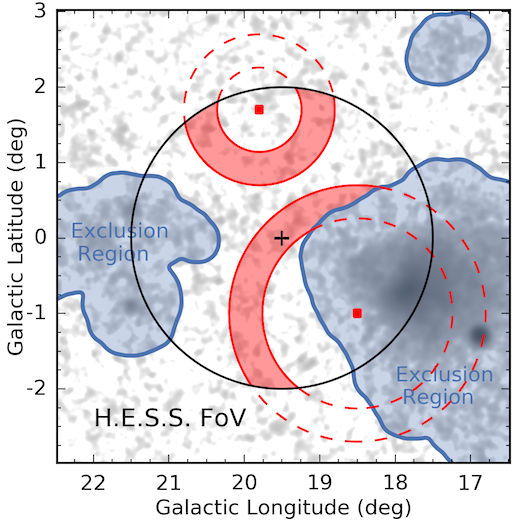# Ring background#

## Overview#

This background estimation technique is used in classical Cherenkov astronomy for 2D image analysis. The working principle is illustrated in Fig. 1. For any given pixel in a counts map the off counts are estimate from a ring centered on the test position with a given fixed radius and width. To improve the estimate, regions with known gamma-ray emission are excluded from measuring the off events. A variation of this methods is given by the “adaptive” ring. In some regions (e.g. in the Galactic plane) a lot of gamma-ray emission has to be excluded and there are not many regions left to estimate the background from. To improve the off statistics in this case, the ring is adaptively enlarged to achieve a defined minimal background efficiency ratio (see Notations). More details on the ring background method can be found in [Berge2007].Fig.1, Illustration of the ring background estimation method, taken from [Abdalla2018].#

To include the classical ring background estimation into a data reduction chain, Gammapy provides the `RingBackgroundMaker` and `AdaptiveRingBackgroundMaker` classed. These classes can only be used for image based data. A given `MapDataset` has to be reduced to a single image by calling `MapDataset.to_image()`

```from gammapy.makers import MapDatasetMaker, RingBackgroundMaker, SafeMaskMaker
from gammapy.datasets import MapDataset
from gammapy.data import DataStore
from gammapy.maps import MapAxis, WcsGeom, Map
from regions import CircleSkyRegion
from astropy import units as u

data_store = DataStore.from_dir("\$GAMMAPY_DATA/hess-dl3-dr1")
observations = data_store.get_observations([23592, 23559])

energy_axis = MapAxis.from_energy_bounds("1 TeV", "10 TeV", nbin=1)
energy_axis_true = MapAxis.from_energy_bounds("0.3 TeV", "30 TeV", nbin=20, per_decade=True, name="energy_true")

geom = WcsGeom.create(skydir=(83.63, 22.01), axes=[energy_axis], width=5, binsz=0.02)

stacked = MapDataset.create(geom, energy_axis_true=energy_axis_true)

maker = MapDatasetMaker()
methods=["aeff-default", "offset-max"], offset_max="2.5 deg"
)

circle = CircleSkyRegion(center=geom.center_skydir, radius=0.2 * u.deg)